# Introduction to the NicheMapR microclimate model

#### 2019-10-08

This vignette provides a detailed tutorial of the Niche Mapper microclimate model as implemented in the package NicheMapR. See the microclimate-model-theory-equations vignette for details on the underlying theory and equations.

## Getting started: working with the micro_global() function

The NicheMapR package includes data and functions for using a global monthly climate database for computing microclimates. This tutorial illustrates the different capabilities of the microclimate model using this database. The vignettes microclimate-IO and microclimate-hourly-example illustrate how to customise the model to work with other sources of data.

### Basic operation: modelling microclimates for the average day of each month

This first example involves the most basic case of running the model to produce 12 days of output, one for each month of the year, without invoking the soil moisture or snow subroutines. In this mode of operation, the model does three iterations of each day, starting with a uniform soil temperature profile and the first two simuulations acting as ‘burn-in’ runs prior to the third and final run which is provided as the final output.

Try running the model for a place of interest to you, as follows:

library(NicheMapR)
longlat <- c(-89.40123, 43.07305) # Madison, Wisconsin, USA
micro <- micro_global(loc = longlat)

The results are stored as a list in the variable micro. The main ones to focus on for now are

• metout The above ground micrometeorological conditions under the minimum specified shade
• shadmet The above ground micrometeorological conditions under the maximum specified shade
• soil Hourly predictions of the soil temperatures under the minimum specified shade
• shadsoil Hourly predictions of the soil temperatures under the maximum specified shade

The first two rows of the output tables metout and shadmet look like this

DOY TIME TALOC TAREF RHLOC RH VLOC VREF SNOWMELT
15 0 -14.13 -13.39 79.22 74.00 0.43 1.96 0
15 60 -14.27 -13.54 82.89 77.48 0.37 1.71 0
POOLDEP PCTWET ZEN SOLR TSKYC DEW FROST SNOWFALL SNOWDEP
0 0 90 0 -26.81 0 0 0 0
0 0 90 0 -26.85 0 0 0 0

and show, for each day of the year and for each hour of the day, a series of aboveground microclimatic conditions as follows:

1. DOY - day of year
2. TIME - time of day (mins)
3. TALOC - air temperature (°C) at local height (specified by ‘Usrhyt’ variable)
4. TAREF - air temperature (°C) at reference height (1.2m)
5. RHLOC - relative humidity (%) at local height (specified by ‘Usrhyt’ variable)
6. RH - relative humidity (%) at reference height (1.2m)
7. VLOC - wind speed (m/s) at local height (specified by ‘Usrhyt’ variable)
8. VREF - wind speed (m/s) at reference height (1.2m)
9. SNOWMELT - snowmelt (mm)
10. POOLDEP - water pooling on surface (mm)
11. PCTWET - soil surface wetness (%)
12. ZEN - zenith angle of sun (degrees - 90° = below the horizon)
13. SOLR - solar radiation (W/m2)
14. TSKYC - sky radiant temperature (°C)
15. DEW - dew presence (0 or 1)
16. FROST - frost presence (0 or 1)
17. SNOWFALL - snow predicted to have fallen (mm)
18. SNOWDEP - predicted snow depth (cm)

Note that for air temperature, relative humidity and wind speed, there’s a ‘reference height’ value and a ‘local height’ value. The reference height value is the height at which the meteorological observations were made that were given to the model as input. The local height values are the microclimatic values at a height determined by the variable Usrhyt, which you can set to be relevant to the organism you are interested in (by default it is 1cm). It should be at the midpoint of the animal or plant’s height.

Check what you got with:

metout<-micro$metout # put the metout result into a variable 'metout' head(metout,24) # show the first 24 rows, i.e. the first day shadmet<-micro$shadmet # put the shadmet result into a variable 'shadmet'
head(shadmet,24) # show the first 24 rows, i.e. the first day

Because the snow model wasn’t flagged to run, there will be zeros in all the snow-related columns.

The first two rows of the output tables soil and shadsoil look like this

DOY TIME D0cm D2.5cm D5cm D10cm D15cm D20cm D30cm D50cm D100cm D200cm
15 0 -15.11 -12.64 -11.31 -10.89 -10.80 -10.87 -11.11 -11.07 -9.92 7.29
15 60 -15.19 -12.95 -11.52 -11.05 -10.88 -10.89 -11.09 -11.06 -9.89 7.29

and show, for each day of the year and for each hour of the day, the soil temperatures as follows:

1. DOY - day of year
2. TIME - time of day (mins)
3. Columns 3-12 D0cm … - soil temperatures at each of the 10 specified depths

Have a look at yours:

soil<-micro$soil # put the soil result into a variable 'soil' head(soil,24) # show the first 24 rows, i.e. the first day shadsoil<-micro$shadsoil # put the metout result into a variable 'shadsoil'
head(shadsoil,24) # show the first 24 rows, i.e. the first day

The 10 different depths used by the model are specified with DEP and by default are DEP=c(0., 2.5, 5., 10., 15., 20., 30., 50., 100., 200.).

There are some example plots of all the variables in the help for the micro_global function. Here is a plot for all the soil temperatures in Madison, Wisconsin in July and December:

require(lattice, quietly = TRUE)
soil <- as.data.frame(micro$soil) # get the soil data minshade <- micro$minshade # get the value for minimum shade
with(subset(soil, DOY==196 | DOY==349), {xyplot(D0cm + D2.5cm + D5cm + D10cm + D15cm + D20cm + D30cm + D50cm + D100cm + D200cm ~ TIME | as.factor(DOY), ylim = c(-20, 70), xlab = "Time of Day (min)", ylab = "Soil Temperature (deg C)", auto.key = list(columns = 5), as.table = TRUE, type = "b", main = paste(minshade,"% shade"))})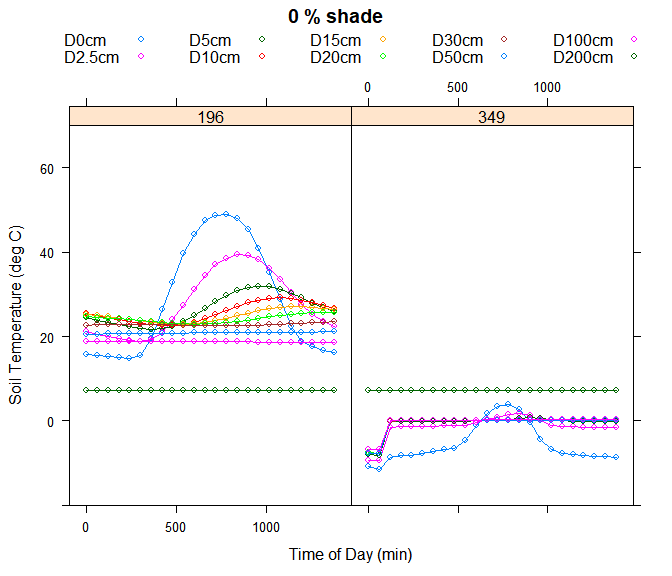Notice how the deeper one goes into the soil, the lower the amplitude of the fluctuations but also that the maximum temperature is reached later in the day. The daily fluctuations have largely disappeared by 50cm and at 200cm the temperature is stable year round. In fact the model assumes the deep soil temperature is the annual mean of the air temperatures, and this is a ‘boundary condition’ in the solution of the soil heat budget.

### Simulating shading by vegetation

By default the model runs twice, for two different levels of shading by vegetation. The particular shade levels can be selected with the parameters maxshade and minshade. Also, the second shade level simulation can be turned off if only one shade level is required with the parameter runshade. The code below runs the model for one shade level only, 50%:

micro <- micro_global(loc = longlat, runshade = 0, minshade = 50)
soil <- as.data.frame(micro$soil) # get the soil data minshade<-micro$minshade # get the value for minimum shade
with(subset(soil, DOY==196 | DOY==349),{xyplot(D0cm + D2.5cm + D5cm + D10cm + D15cm + D20cm + D30cm + D50cm + D100cm + D200cm ~ TIME | as.factor(DOY), ylim = c(-20, 70), xlab = "Time of Day (min)", ylab = "Soil Temperature (deg C)", auto.key=list(columns = 5), as.table = TRUE, type = "b", main = paste(minshade, "% shade"))})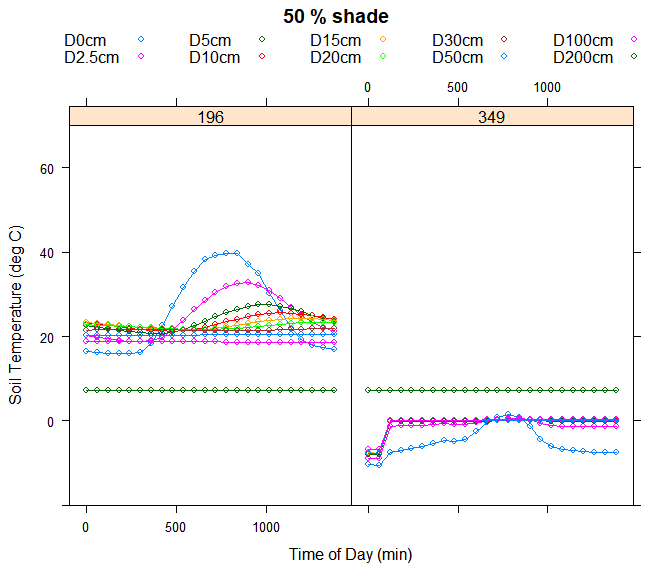Notice how, the effect of shade is to not only reduce the daytime soil temperatures, but also to increase the nighttime temperatures. This is due to the increased levels of infrared radiation reaching the ground surface because the shading vegetation is assumed to be at the reference height air temperature while the ‘sky temperature’ is typically much colder, depending on the level of cloud cover (have a look at the variable Tsky in the metout and shadmet outputs).

### Simulating terrain effects - slope, aspect, hillshade

Slope and aspect have strong effects on microclimates through the effects of direct solar radiation. The default setting is flat ground in the NicheMapR microclimate model. Here is the effect of a 45 degree southerly slope on the 0% shade simulation:

micro <- micro_global(loc = longlat, runshade = 0, minshade = 0, slope = 45, aspect = 180)
soil <- as.data.frame(micro$soil) # get the soil data minshade <- micro$minshade # get the value for minimum shade
with(subset(soil, DOY==196 | DOY==349), {xyplot(D0cm + D2.5cm + D5cm + D10cm + D15cm + D20cm + D30cm + D50cm + D100cm + D200cm ~ TIME | as.factor(DOY), ylim = c(-20, 70), xlab = "Time of Day (min)", ylab = "Soil Temperature (deg C)", auto.key=list(columns = 5), as.table = TRUE, type = "b", main = paste(minshade, "% shade, 45 degree slope, 180 degrees aspect"))})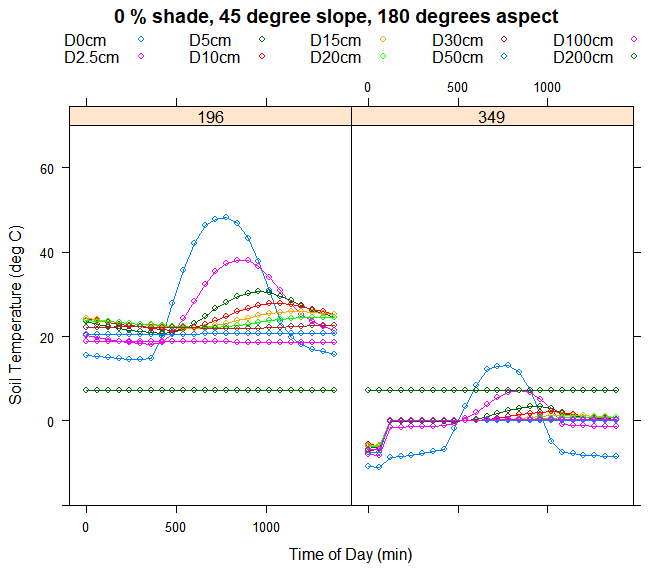The strongest effect is on the December soil temperatures.

Hillshade also has a strong effect on microclimates. Gullies and gorges receive a shorter window of direct solar radiation and have a smaller ‘view’ of the sky, and so don’t cool down as much at night. Horizon angles can be specified to simulated these kinds of effects by supplying in the parameter hori a vector of 24 horizon angles (angle to nearest hill/cliff) starting due north and continuing clockwise in 15 degree intervals. Here is an example for a steep-sided gully running north-south:

micro <- micro_global(loc = longlat, runshade = 0, minshade = 0, hori = c(0, 0, 65, 65, 65, 65, 65, 65, 65, 65, 65, 65, 0, 0, 65, 65, 65, 65, 65, 65, 65, 65, 65, 65))
soil <- as.data.frame(micro$soil) # get the soil data minshade <- micro$minshade # get the value for minimum shade
with(subset(soil,DOY==196 | DOY==349),{xyplot(D0cm + D2.5cm + D5cm + D10cm + D15cm + D20cm + D30cm + D50cm + D100cm + D200cm ~ TIME | as.factor(DOY), ylim = c(-20, 70), xlab = "Time of Day (min)", ylab = "Soil Temperature (deg C)", auto.key = list(columns = 5), as.table = TRUE, type = "b", main=paste(minshade,"% shade, north-south gully"))})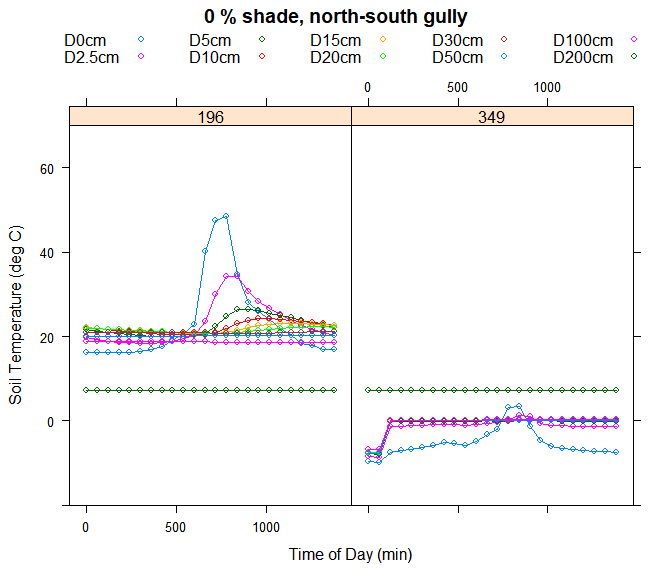### Changing the soil properties

Another important influence on microclimatic conditions is the substrate type. The substrate solar reflectivity can be changed with the parameter REFL and the emissivity with the parameterSLE (both range from 0-1).

Soil thermal properties are also importance. Soil comprises a mixture of minerals, air and water. The density (Mg/m3), thermal conductivity (W/mK) and specific heat capacity (J/kg-K) of the mineral component are specified by the parameters Density, Thcond and SpecHeat, respectively. The bulk density determines the proportion of the soil volume that is air, parameter BulkDensity (Mg/m3). The overall soil conductivity, specific heat and density values are then adjusted for bulk density and soil moisture (see below), as well as for soil temperature, using themethods described in Campbell et al. (1994, equations 8, 9) and Campbell and Norman (Campbell & Norman 1998, equations 8.13, 8.17, 8.20 and data in Tables 8.2 and 9.1). All these properites can be specified separately for each soil node in the model.

The default values are typical for soil minerals as described in Campbell and Norman (1998) Table 8.2, but an ‘A-horizon’ layer for the first node, reflecting properties of organic matter, is also simulated (see Kearney et al. 2014). This can be turned off by specifying cap = 0.

For simulating rock, BulkDensity should be set the same as Density and this can be easily set in micro_global by setting soiltype to value 0 (which also sets cap = 0):

micro <- micro_global(runshade = 0, minshade = 0, soiltype = 0)
soil <- as.data.frame(micro$soil) # get the soil data minshade <- micro$minshade # get the value for minimum shade
with(subset(soil, DOY==196 | DOY==349), {xyplot(D0cm + D2.5cm + D5cm + D10cm + D15cm + D20cm + D30cm + D50cm + D100cm + D200cm ~ TIME | as.factor(DOY), ylim = c(-20, 70), xlab = "Time of Day (min)", ylab = "Soil Temperature (deg C)",  auto.key = list(columns = 5), as.table = TRUE, type = "b", main = paste(minshade, "% shade, rock substrate"))})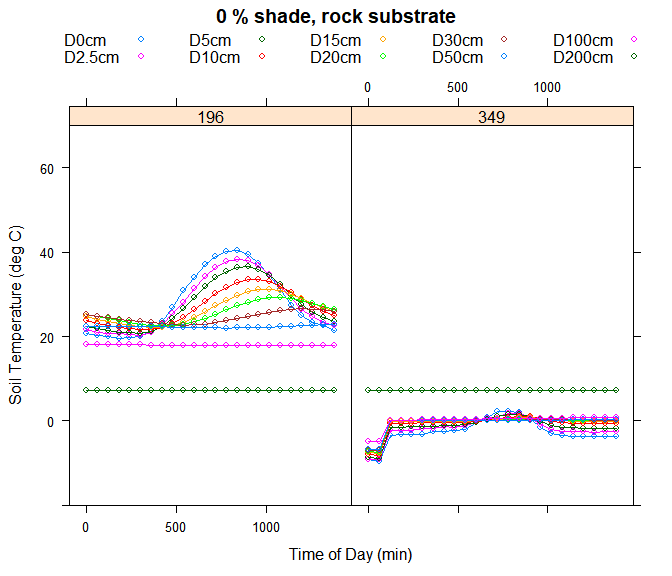Notice how the heat penetrates more deeply into the rock, producing a gentler temperature gradient with depth, cooler near-surface temperatures during the day and warmer near-surface temperatures at night.

The texture of the soil, i.e. the proportion of silt, sand and clay and associated particle size distributions, has a powerful influence on soil moisture and is discussed further in the next section.

### Soil moisture

The microclimate model has the option of either specifing soil moisture or modelling it from first principles. The micro_global function by default monthly soil moisture estimates obtained from the Climate Prediction Center(NOAA/OAR/ESRL PSD, Boulder, Colorado, USA, http://www.esrl.noaa.gov/psd/).

The model will compute soil moisture when the runmoist parameter = 1. This invokes an implementation of Campbell’s (1985) Program 11.1 for simulating depth-specific soil moisture, water potential and humidity gradients in the presence of vegetation. It uses matric potential as the dependent variable (rather than matric flux potential) and as a consequence is most computationally efficient when soil is relatively dry (it may significantly extend run times in wet environments).

The soil moisture calculations are made at the 10 user-specified nodes (parameter DEP) and also at the midpoints between them, but are only reported for the 10 nodes in DEP. The user input variables specific to this module include, for each of these 19 nodes, the Campbell (or Clapp and Hornberger) b exponent (BB), the air entry potential PE (J/kg), the hydraulic conductivity KS (kg s m-3), the bulk density BD (Mg m-3), the density DD (Mg m-3) the root density L (m m-3) and the leaf area index LAI. All other model parameters are fixed at those suggested by Campbell (1985). The model has been tested against detailed observations across Australia with favourable results (Kearney et al. in prep).

Try running the model for your site with the soil moisture model turned on:

micro <- micro_global(loc = longlat, runmoist = 1)

The output tables soilmoist and shadmoist, which previously were zero, will now have soil moisture values (m3/m3) in them:

DOY TIME WC0cm WC2.5cm WC5cm WC10cm WC15cm WC20cm WC30cm
15 0 0.2889611 0.1314211 0.1510331 0.1987071 0.2450959 0.2944447 0.2999247
15 60 0.2512338 0.1690480 0.1531151 0.1976396 0.2428378 0.2882834 0.2996341
WC50cm WC100cm WC200cm
0.2988647 0.2999463 0.4921875
0.2986667 0.2999367 0.4921875

The model also gives you the soil water potential (the water-equivalent to temperature and the driving force for water flow, e.g. into or out of water-permeable eggs or skin) in the outputs soilpot and shadpot:

DOY TIME PT0cm PT2.5cm PT5cm PT10cm PT15cm PT20cm PT30cm
15 0 -12.08379 -418.7811 -223.9522 -65.16584 -25.34944 -11.10359 -10.21940
15 60 -22.67928 -134.8760 -210.5715 -66.76484 -26.42756 -12.21214 -10.26407
PT50cm PT100cm PT200cm
-10.38352 -10.21608 -1.1
-10.41453 -10.21756 -1.1

Finally, the soil humidity is also computed (outputs humid and shadhumid):

DOY TIME RH0cm RH2.5cm RH5cm RH10cm RH15cm RH20cm RH30cm
15 0 0.9998985 0.9965222 0.9981479 0.9994614 0.9997905 0.9999082 0.9999155
15 60 0.9998095 0.9988763 0.9982569 0.9994479 0.9997815 0.9998990 0.9999151
RH50cm RH100cm RH200cm
0.9999141 0.9999159 0.9999159
0.9999139 0.9999159 0.9999159

Note that soil humidity is typically close to saturation, even in ‘dry’ soils. The situation in a burrow may lower than this but still much higher than aboveground conditions (see Schmidt-Nielsen & Schmidt-Nielsen. 1950).

Plotting the results for soil moisture through time across the year:

soilmoist <- as.data.frame(micro$soilmoist) # get the minimum shade soil moisture output minshade <- micro$minshade # get the value for minimum shade
# append dates
days <- rep(seq(1, 12), 24)
days <- days[order(days)]
dates <- days + soilmoist$TIME / 60 / 24 - 1 # dates for hourly output soilmoist<-cbind(dates, soilmoist) for(i in 1:10){ if(i == 1){ plot(soilmoist[, i + 3] ~ soilmoist[, 1], ylim = c(0, 0.5), xlab = "Month", ylab = "Soil Moisture (% vol)", col = i, type = "l", main = paste("soil moisture, ", minshade, "% shade")) }else{ points(soilmoist[, i + 3] ~ soilmoist[, 1], col = i, type = "l") } }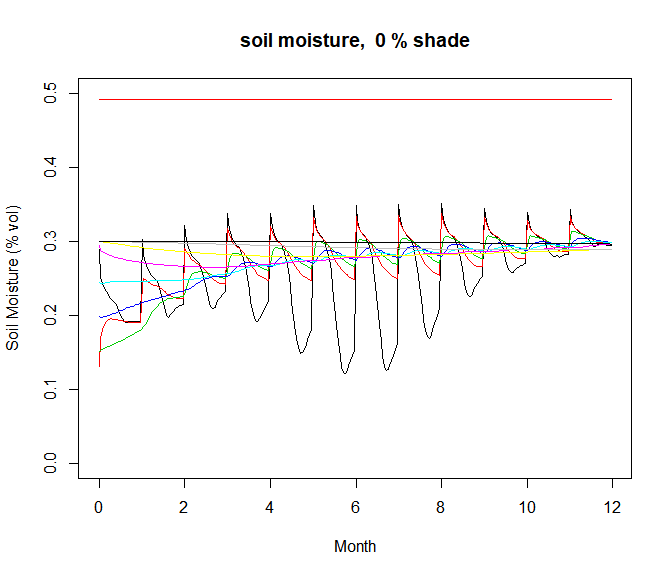By default the model uses soiltype = 4, where Rock = 0, sand = 1, loamy sand = 2, sandy loam = 3, loam = 4, silt loam = 5, sandy clay loam = 6, clay loam = 7, silt clay loam = 8, sandy clay = 9, silty clay = 10, clay = 11, based on Campbell and Norman 1990 Table 9.1. One can also manually set the soil properites by setting soiltype = 12, upon which the model will use the user-specified values of PE, KS, BB, DD and DD. Rerunning with clay as the soil type: micro<-micro_global(loc = longlat, runmoist = 1, soiltype = 11) soilmoist<-as.data.frame(micro$soilmoist) # get the minimum shade soil moisture output
minshade<-micro$minshade # get the value for minimum shade # append dates days<-rep(seq(1,12),24) days<-days[order(days)] dates<-days+soilmoist$TIME/60/24-1 # dates for hourly output
soilmoist<-cbind(dates,soilmoist)
for(i in 1:10){
if(i==1){
plot(soilmoist[,i+3]~soilmoist[,1], ylim=c(0,0.5),xlab = "Month", ylab = "Soil Moisture (%
vol)",col=i,type = "l",main=paste("soil moisture, ",minshade,"% shade"))
}else{
points(soilmoist[,i+3]~soilmoist[,1] ,col=i,type = "l")
}
}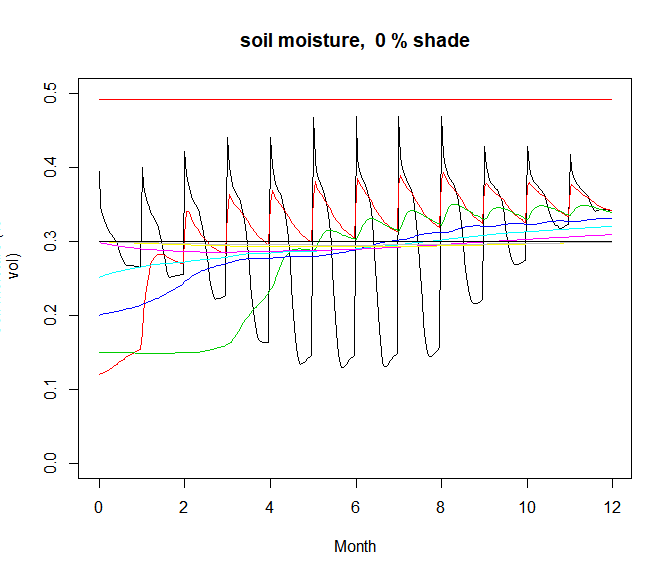we see strong changes in the soil moisture values, with the fine-grained clay particles holding on more strongly to the water resulting in higher soil water content. Also very apparent in these plots is that the model was not reaching steady state soil moisture values with only 3 iterations of each day. This can be resolved by running the model for longer periods, as discussed in the next section.

### Running at finer time intervals and for longer time periods

It is possible to run the model for multiple years by changing the nyears parameter. This can be useful when running the soil moisture and snow subroutines to allow annual cycles to stabilise. To illustrate, if we run the last example in the soil moisture section for five years, the soil moisture at all but the deepest levels have started to reach steady state:

nyears<-5
micro<-micro_global(loc = longlat, runmoist = 1, soiltype = 11, nyears = nyears)
soilmoist<-as.data.frame(micro$soilmoist) # get the minimum shade soil moisture output minshade<-micro$minshade # get the value for minimum shade
# append dates
days<-rep(seq(1,12*nyears)/12,24)
days<-days[order(days)]
dates<-days+soilmoist$TIME/60/24/(12) # dates for hourly output soilmoist<-cbind(dates,soilmoist) for(i in 1:10){ if(i==1){ plot(soilmoist[,i+3]~soilmoist[,1], ylim=c(0,0.5),xlab = "Year", ylab = "Soil Moisture (% vol)",col=i,type = "l",main=paste("soil moisture, ",minshade,"% shade")) }else{ points(soilmoist[,i+3]~soilmoist[,1],col=i,type = "l") } }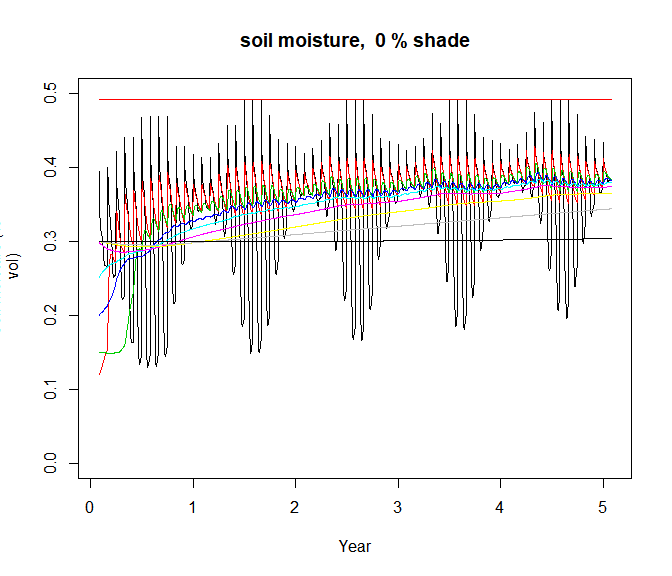It is also possible to run the model for more frequent intervals than once per month by changing the parameter timeinterval. If timeinterval is set to something less than 365, then the model runs as described in the previous section with three iterations per day, and uses the ‘spline’ function to interpolate the climate data from monthly to the chosen time interval. If ‘timeinterval’ is set to the maximum value of 365, it will apply the three iterations to the first day of the simulation to get started and then, from that day on, use the previous day’s conditions as the initial state for the next day’s conditions. Here is an example running the model for 365 days and plotting soil moisture: timeinterval <- 365 micro <- micro_global(loc = longlat, runmoist = 1, soiltype = 11, timeinterval = timeinterval) soilmoist <- as.data.frame(micro$soilmoist)  # get the minimum shade soil moisture output
minshade <- micro$minshade # get the value for minimum shade # append dates days <- rep(seq(1, timeinterval), 24) days <- days[order(days)] dates <- days + soilmoist$TIME/60/24 - 1  # dates for hourly output
soilmoist <- cbind(dates, soilmoist)
for (i in 1:10) {
if (i == 1) {
plot(soilmoist[, i + 3] ~ soilmoist[, 1], ylim = c(0, 0.5), xlab = "Day of Year", ylab = "Soil Moisture (% vol)", col = i, type = "l", main = paste("soil moisture, ", minshade, "% shade"))
} else {
points(soilmoist[, i + 3] ~ soilmoist[, 1], col = i, type = "l")
}
}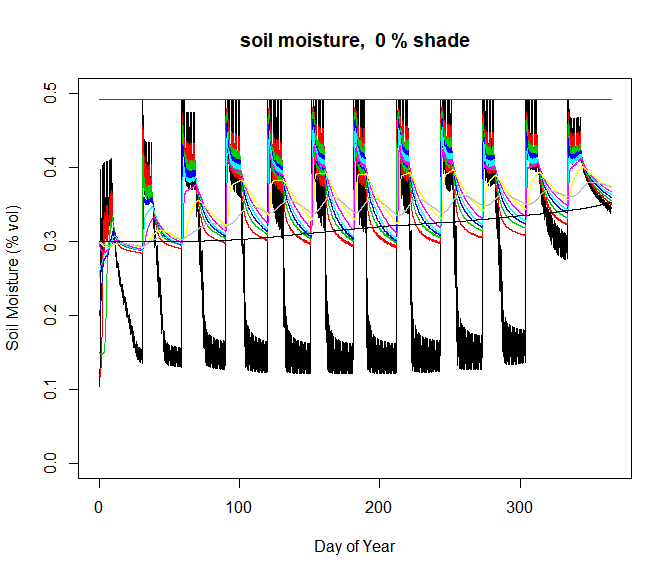Here are the associated soil temperatures:

soil <- as.data.frame(micro$soil) # get the minimum shade soil temperature output minshade <- micro$minshade  # get the value for minimum shade
# append dates
days <- rep(seq(1, timeinterval), 24)
days <- days[order(days)]
dates <- days + soil$TIME/60/24 - 1 # dates for hourly output soil <- cbind(dates, soil) for (i in 1:10) { if (i == 1) { plot(soil[, i + 3] ~ soil[, 1], ylim = c(-20, 70), xlab = "Day of Year", ylab = "Soil Temperature (deg C)", col = i, type = "l", main = paste("soil temperature, ", minshade, "% shade")) } else { points(soil[, i + 3] ~ soil[, 1], col = i, type = "l") } }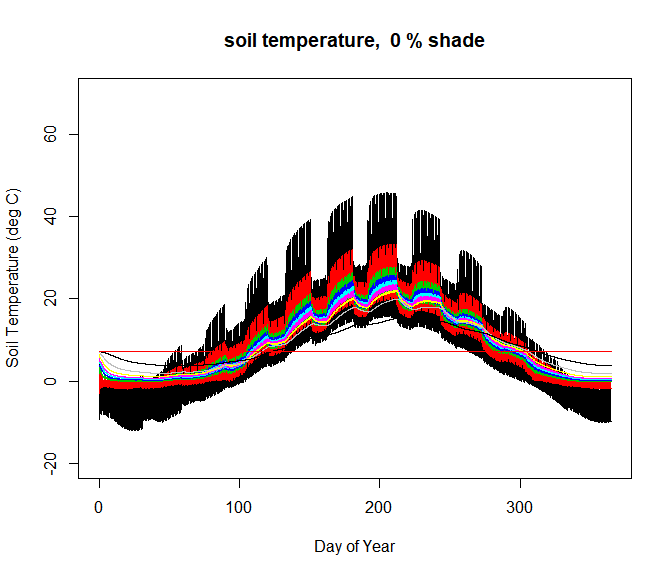### Snow Snow has a powerful influence on soil temperatures and can be simulated in the NicheMapR microclimate model by setting the parameter snowmodel = 1. This invokes an algorithm that builds up to 8 nodes of snow above the surface as a function of incoming precipitation and losses due to melting. The model incorporates a declining albedo with time since snow and has five user-controlled parameters: snowtemp - the air temperature (°C) at which rain falls as snow, snowdens (Mg/m3) - the density of the snow, snowmelt (-) - the proportion of calculated snow melt that doesn’t refreeze into the snowpack, rainmelt (-) a parameter in an equation from Anderson’s SNOW17 model (http://www.nws.noaa.gov/oh/hrl/nwsrfs/users_manual/part2/_pdf/22snow17.pdf) that melts snow with rainfall as a function of air temperature. The snow density can also be made to vary as a linear function of day of year by providing a slope and intercept as the variable densfun, which is only used if non-zero. There is also a parameter rainfrac which controls the fraction of rain that falls on the first day of the month, with 0 meaning rain falls evenly. This parameter allows something other than an even intensity of rainfall when interpolating the montly rainfall data. This snow model has been tested with favourable results in the Australian Alps and through the USA (Kearney et al. in prep). Here is the consequence of invoking the snow model for soil temperature predictions at Madison, Wisconsin as well as the snow depth and snowfall predictions (running for 2 years to get a steady periodic and plotting only the 2nd year): timeinterval <- 365 nyears <- 2 # running two years, first one acting as a 'burn in' year and discarded micro <- micro_global(loc = longlat, runmoist = 1, snowmodel = 1, timeinterval = timeinterval, nyears = 2) soil <- as.data.frame(micro$soil)[(365 * 24 + 1):(365 * 24 * nyears), ]  # get the minimum shade soil temperature output, discarding the first year
metout <- as.data.frame(micro$metout)[(365 * 24 + 1):(365 * 24 * nyears), ] # get the minimum shade above ground conditions, discarding the first year minshade <- micro$minshade  # get the value for minimum shade
# append dates
days <- rep(seq(366, timeinterval * nyears), 24)
days <- days[order(days)]
dates <- days + soil$TIME / 60 / 24 - 1 # dates for hourly output soil <- cbind(dates, soil) metout <- cbind(dates, metout) for (i in 1:10) { if (i == 1) { plot(soil[, i + 3] ~ soil[, 1], ylim = c(-20, 70), xlab = "Day of Year", ylab = "Soil Temperature (deg C)", col = i, type = "l", main = paste("soil temperature, ", minshade, "% shade")) } else { points(soil[, i + 3] ~ soil[, 1], col = i, type = "l") } }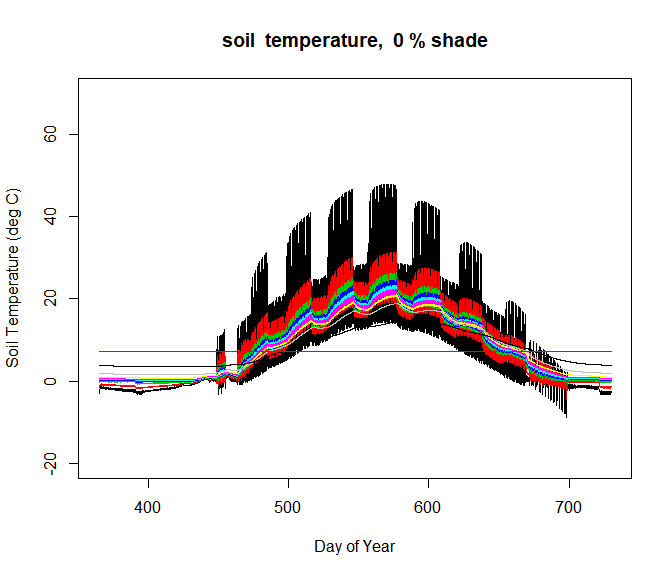plot(metout$SNOWDEP ~ metout$dates, xlab = "Time of Day (min)", ylab = "snow depth, cm / snow fall, mm", type = "h", main = paste("snow depth (cm) and snow fall (mm) ", minshade, "% shade", sep = ""), col = "light blue") points(metout$SNOWFALL ~ metout$dates, xlab = "Time of Day (min)", type = "h", col = "blue")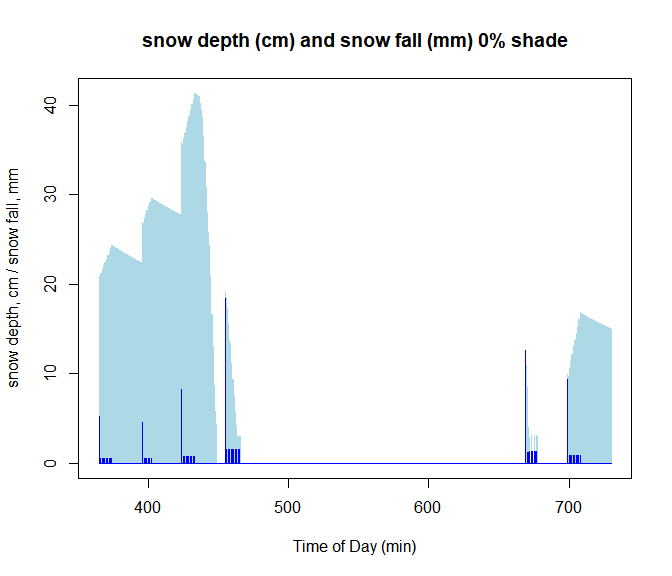### The intertidal zone The final application illustrated in this vignette concerns predicting the complex environment of the intertidal zone, where tidal influences impose strong effects on intertidal zones in a manner out of phase with the diurnal cycle. When the argument shore = 1, the microclimate model attempts to simulate such influences through a user-specified matrix tides of hourly values for 1) tide presence, 2) sea water temperature and 3) presence of wave splash. If the tide is present in a given hour, the model simplifies to heat exchange only by convection and conduction, with an arbitrarily large ‘wind speed’ imposed to strengthen the coupling of the sea water to the substrate. The effect of the wave splash vector is to make the surface wet and so exchange heat by evaporation. This module is currently untested. An example of what is possible is provided below, and illustrates what might happen on a rocky section of the shore of Lake Mendota in Madison: nyears<-1 timeinterval<-365 # if you have data on tides for a shoreline, use the code below to specify the hours the tide is in, the water temperature and wave splash tides<-matrix(data = 0., nrow = 24*timeinterval*nyears, ncol = 3) # make an empty matrix tides[,2]<-5 # set a constant sea water temperature tides[,3]<-0 # zero the wave splash column tides[12,3]<-90 # make the 12th value (hour 12 on 1st day) get a spash, resulting in the rock evaporating as if it is 90% wetted mocktides<-rep(c(rep(0,12),rep(1,13)),timeinterval*nyears) # made a sequence of tides, where 1 means tide is in and 2 means out, and offset it to 24 hour cycle mocktides<-mocktides[1:8760] # subset it to a year long of 24 hour tides tides[1:(timeinterval*nyears*24),1]<-mocktides # put the mock tides in the tides vector, column 1 micro<-micro_global(loc=longlat, shore = 1, soiltype = 0, timeinterval = timeinterval, nyears = nyears, tides = tides) soil<-as.data.frame(micro$soil) # get the minimum shade soil temperature output first year
minshade<-micro$minshade # get the value for minimum shade # append dates days<-rep(seq(1,timeinterval),24) days<-days[order(days)] dates<-days+soil$TIME/60/24-1 # dates for hourly output
soil<-cbind(dates,soil)
for(i in 1:10){
if(i==1){
plot(soil[,i+3]~soil[,1], ylim=c(-20,70),xlab = "Day of Year", ylab = "Soil Temperature (deg
C)",col=i,type = "l",main=paste("soil temperature, ",minshade,"% shade"))
}else{
points(soil[,i+3]~soil[,1], col=i,type = "l")
}
}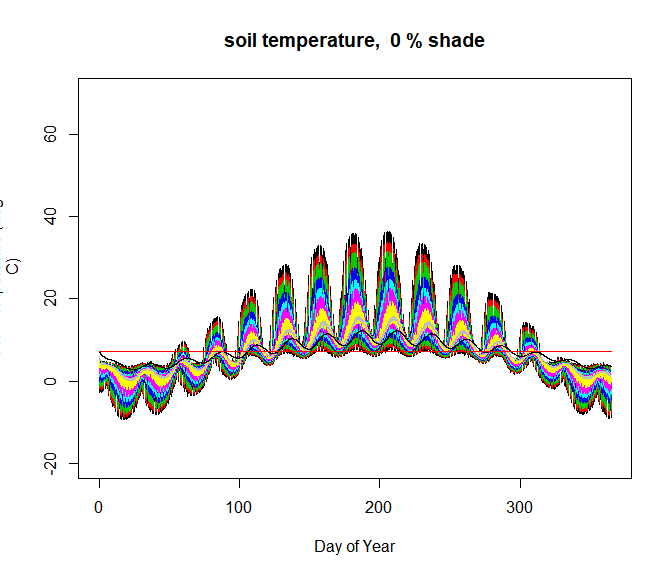NOTE specifying too disparate a sea water temperature from the substrate temperature may result in the model crashing!

### Other parameters

In the help for the micro_global function you’ll find information on a number of other parameters that may be of use for your application. These allow control of the eccentricity of the earth’s orbit EC (if you want to go way back into the past), changes in the timing of daily maxima and minima for air temperature, cloud cover, wind speed and relative humidity TIMINS and TIMAXS, the optical effects of the amount of water in the upper atmosphere CMH2O, whether to apply the Global Aerosol Data Set (package GADS) to determine solar attenuation due to dust etc. in the atmosphere rungads, whether the model outputs csv files writecsv or csv files of all inputs writeinput (mainly used for the maintainers of the program when testing the Fortran code).